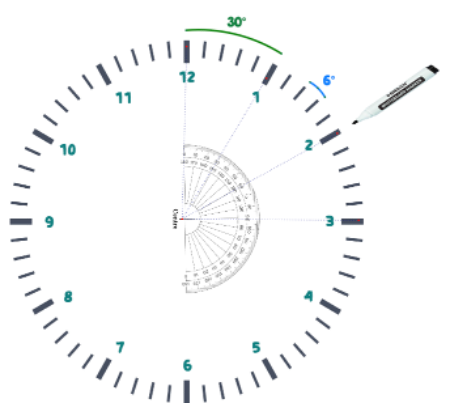Ara
•Eda Aydemir

# Create Your Own Math Clock

### ETKİNLİĞİN TÜRKÇESİNİ PDF OLARAK İNDİREBİLİRSİNİZ.

Unit: Mathematics of Timekeeping

Activity: Create your own math clock

Our goal is to write the numbers to the proper places of the blank clock.

We can explore many mathematical concepts by using this activity.

For thousands of years, devices have been used to measure and keep track of time. Sundials, pendulum clocks, hour glasses are used till the invention of mechanical clocks. Today atomic clocks are used to tell the time precisely.

There is a very nice article and a video on “A History Of Timekeeping” Page by British Museum.

You may also check the History of Timekeeping Devices on Wiki

### Essential Questions:

Why 1 hour is 60 minutes?

What are the different number systems in human history (Babylonians to start with) that uses the sexagesimal counting system?

• Watch the video from Numberphile.

• The current sexagesimal system of time measurement dates to approximately 2000 BCE from the Sumerians.

### STEAM Connections:

History of timekeeping

Please watch the "A Brief History of Timekeeping" by SciShow.

And read the article on Britannica for Kids or the article “A Chronicle Of Timekeeping” by Scientific American

Search about different types of clocks;

Science;Invention of the mechanical clocks

Mechanics of a clock – ready-to-use mechanism.

For the image and explanations, please check Britannica for Kids.

A blank wall clock### Create Your Own Math Clock

CONCEPT #1: FACTORS AND DIVISORS

If you can divide a number A by a number B, without remainder, we say that B is a factor (or divisor) of A, and that A is a multiple of B.

Factors always appear in pairs such as

12 = 1 x 12

12 = 2 x 6

12 = 3 x 4

1 and 12, 2 and 6, 3 and 4 are called the factor pairs. So, the whole list of factors of 12 is {1, 2, 3, 4, 6, 12}

The factors of 60 are _________1, 2, 3, 4, 5, 6, 10, 12, 15, 20, 30 and 60.

The total number of factors is of 60 is ___12

CONCEPT #2: DIVISION AND FRACTIONS

We can define the non-whole number quantities by using fractions and decimals. For instance, a half is represented as ½ whereas a quarter is represented as ¼.

In fact, most of the numbers can be represented as fractions.

0.2= 1/5

0.333.. =1/3

0.142856.. = 1/7

1 = 1/1 = 2/2 =3/3 …

1.5= 3/2 =6/4= 15/10 ..

2= 2/1 = 4/2 = 6/3 ..

When we say half an hour it means you have ___30 mins.

A quarter of an hour is only ____ 15 mins.

So half past ten: ____ 10:30

A quarter past three: _____ 3:15

A quarter to nine: _____8:45

CONCEPT #3: ANGLES

We need to divide the blank circular clock into 12 equal parts to be able to insert the numbers.

But how we can divide a circle into 12 equal pieces?

It is time to explore angles.

What are the types of angles?

______________So we have a _____ 360 degrees angle to divide by ____ 12

It means, the circle will have numbers to represent hours in every _____ 30 degrees.

There are 60 minutes in every hour. So the minute-hand needs have _____60 stops. Since 360 degrees : 60 = 6, we need to put a little mark on every_____ 6 degrees for the stops of the minute hand.

To be able to measure any given angle or draw an angle with a given measurement we need to use the _________ protractor.

Angle Measurement is a very important skill for all of us to grasp in early ages. If you need, lease watch the angle measurement video on YouTube “Drawing Angles With a Protractor”.

Let’s start putting a mark in every 6 degrees.

Do not worry we are going to do this only 60 times.

Do not forget to insert hours in every 30 degrees.CONCEPT #4: CIRCLES

Up to now, we have explored many concepts related with circles.

To start with, we can figure out that all the points we have inserted on the circle are equidistant to its center.

The set of all equi-distant points from a given point is called a _____ circle. The place we put our protractor to measure and draw the angles- which is exactly in the middle of the circle is called the ______ center of the circle. The hour marks are on the ___ arc of the circle. If we connect the center of the clock where we are going to insert the minute and hour hand and any mark on the arc, that segment is called the _____radius.

Twice the radius is called _____ diameter. Diameter can help you to figure out the dimensions of your clock. The clock I have has a diameter of 24 cm. So on the wall, if I find a square 24 by 24, my clock will definitely fit in it.

Each angle we measure is called the ______ central angle.

These terms will also help us to communicate better.

If you want to color your clock, you may need to calculate the area of the clock to see how much paint you will need. The length of the radius helps us here. The area of any circle is π times the square of its radius. To make a rough estimate, you may multiply the square of your clock’s radius by 3.

When we insert the battery in, the clock starts ticking. That moment is the one that we can start talking about time measurement and all related problems.

We can definitely raise this to make a fancy mathematical clock. Pick a number or use the numbers of your birthday and try to express 1- 12 in terms of the number(s) you choose. You can use all the operations you know.

Mind the order of operation!3 görüntüleme

Hepsini Gör